# Hardware Analysis and Synthesis

## 1 OBJECTIVES

The experimental goal of this lab is for your team to determine if a robot design meets minimum standards for accuracy and precision. To do this your team will build and program a robot, and test it against the provided EG1003 standard. All your results must be tabulated accurately.

Together, we will define the concept of product evaluation, demonstrate the importance of accurate measurements, and show how they relate to both precision and accuracy. Once you have completed your lab work, you will be able to describe the method of troubleshooting you developed.

## 2 OVERVIEW

Your team has been hired by an engineering firm to evaluate a robotics design. The Robotics kit provided has a manufacturer's suggested retail price of \$249.99. The firm has specified the robot design they would like you to build and test. Your assignment is to use the kit to test the specified design, describe what works and list the improvements that should be made to the robot design you built. The price of the kit should be factored into the overall product evaluation. What other factors do engineers consider when they evaluate products?

Product evaluation is a common task for an engineer. Sometimes the product you evaluate will be one your team built, and sometimes you will be analyzing a competitor's design. In either case, you will need to decide on a standard (an accepted value), determine an average, and calculate accuracy and precision.1

Professional engineers must be sure of standards. For the purpose of this lab, the EG1003 Standard that you will be testing to is 80% precision and accuracy. This means that the product you will be testing has to pass 80% of the tests that it undergoes. Some projects may require a degree of accuracy to a tenth of an inch. Attempting to make the project accurate to the hundredth might be unnecessary and difficult to control. It is up to you to verify that your solutions are accurate based on the standard for your design project. 1

When taking measurements, the first step is to determine an average.In this equation, AP is the average, P1, P2, P3, … are the results of the tests and N is the total number of tests to be performed.

The average is then compared to the standard. Accuracy is a variable you must always consider. The degree of accuracy required will allow you to verify your results. Engineers often work on projects that affect people's safety. Therefore, it is critical that the solutions to the problems you will solve as technical professionals are accurate.

The comparison of the average to the standard is called accuracy, defined as Acc in the following equation:

Acc = |PS - AP|

In this equation, PS is the standard value, and AP is the value you measured. Accuracy is always a positive number, so we take the absolute value in case PS is less than AP.

Precision is the repeatability of a result, or how close the results are to each other, defined as Prec in the following equation:

Prec = |Phigh - Plow|

In this equation, Prec is Precision, Phigh is the highest data value and Plow is the lowest data value. Like accuracy, precision is always a positive number, so we use an absolute value here as well.

A product test can be accurate but imprecise, or precise but inaccurate. The goal is always the combination of accuracy and precision. In order for engineers to make a recommendation based on experimental test data, it is important to determine how a device measures against a standard. Engineers need these benchmarks to determine the quality of a product.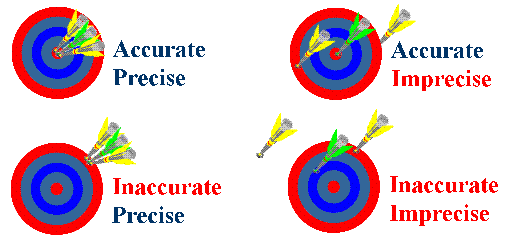By determining the percent accuracy of an experiment, and comparing this percentage to a standard, you can determine, based on experimental evidence, whether the prototype has passed or failed. The equation for percent accuracy is: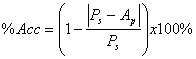In this equation, %Acc is the percent accuracy, PS is the standard value, and AP is the value you measured.

Percent accuracy allows an engineer to determine how precise a device is compared to the standard. The equation for percent precision is:

%Prec = 100e-BP

In this equation, %Prec is the percent precision, B is the exponential decay factor, and P precision you measured.

Calculating percent accuracy and percent precision and then comparing your results to a standard will allow you to determine the quality of your prototype.

### Individual Lab Report

Follow the lab report guidelines laid out in the page called Specifications for Writing Your Lab Reports in the Technical Communication section of this manual. As you write, the following discussion points should be addressed in the appropriate section of your lab report:

• Discuss product evaluation and the need for it.
• Explain the importance of testing.
• Discuss standard, average, accuracy, and precision.
• Explain the importance of designing to a standard.
• Discuss the RoboLab kit and software used.
• Assess the techniques used in the testing process.
• Discuss better testing methods for the robot design.
• Make a final evaluation of the product: should it go into production? Describe its accuracy and precision.
• Discuss the importance of % precision and % accuracy in determining product quality.

### Team PowerPoint Presentation

Follow the presentation guidelines laid out in the page called EG1003 Lab Presentation Format in the Introduction to Technical Presentations section of this manual. When you are preparing your presentation, consider the following points:

• Based on the test results, what is your determination for the product's marketability?
• If determinations were negative, what improvements could be made?
• Why is product evaluation important to you?

## 4 MATERIALS AND EQUIPMENT

• Mindstorms Kit
• Computer with Mindstorms Software
• Protractor and Ruler

## 5 PROCEDURE

### Problem Statement

You have been hired by an engineering firm to build and evaluate a robotics design by building a specified robot design. You have been asked to test the robot design and to make suggestions for design improvements.

1. Build a robot according to the lab handout provided by your TA.
2. Warning: Do not disassemble the robot when you finish. You will use it again in the next lab.

3. Create a program in Mindstorms:
1. Start the Mindstorms Program. On the welcome screen type in a name for your program and press Go.
2.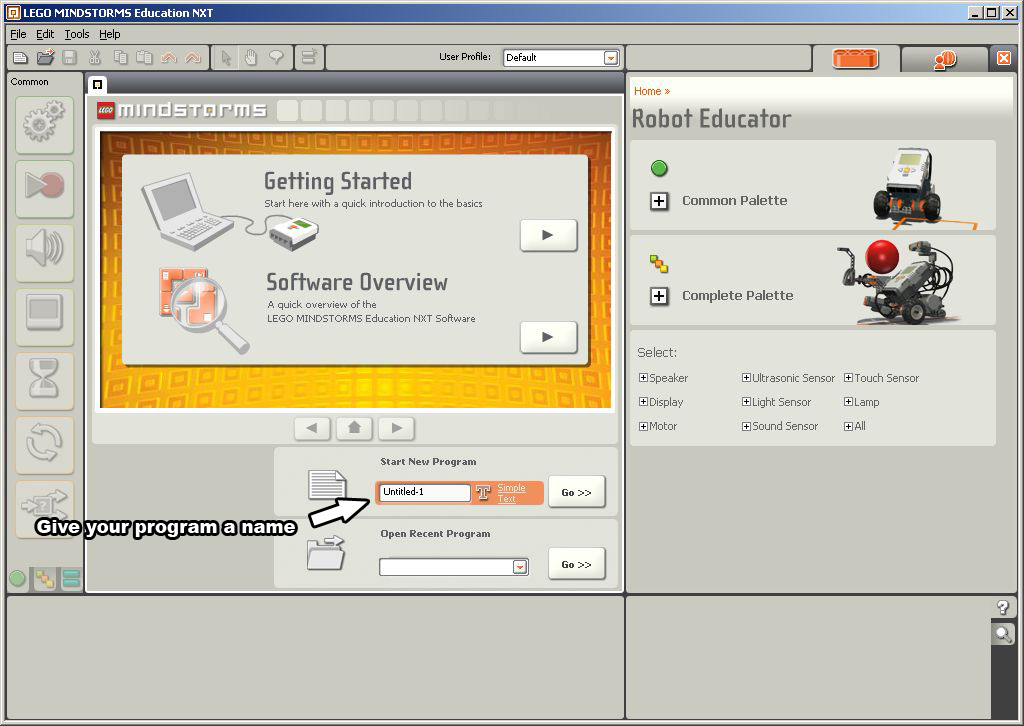3. Click on Complete Pallete to see all the programming icons.
Turn off Robot Educator by clicking the small X.
4.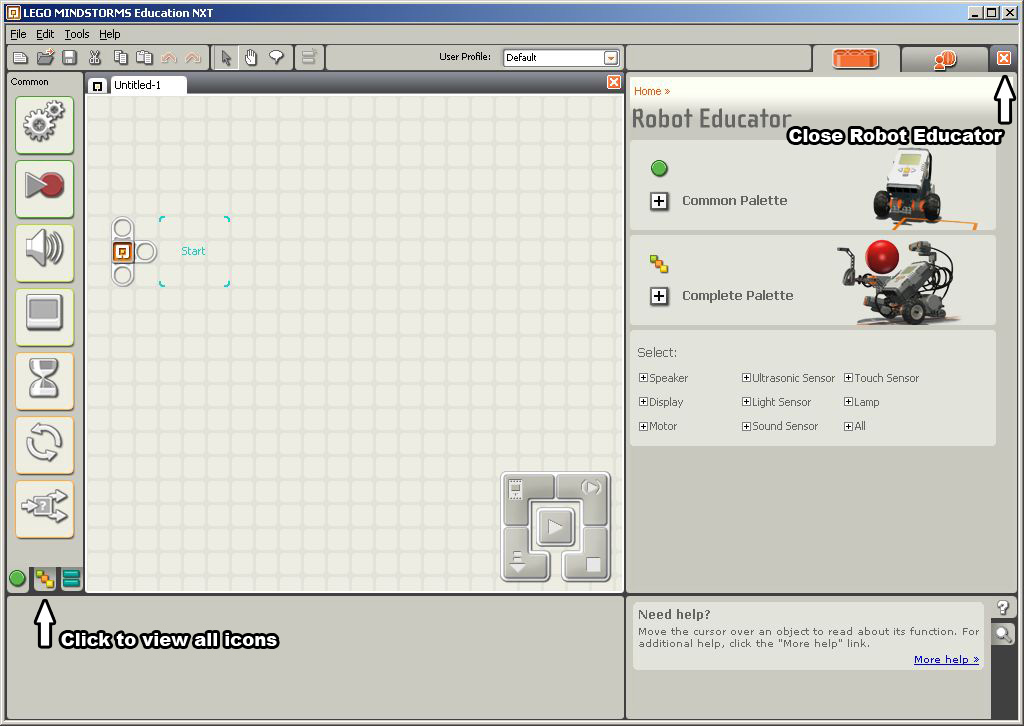5. Your screen should look like this. You are now ready to write a program.
6.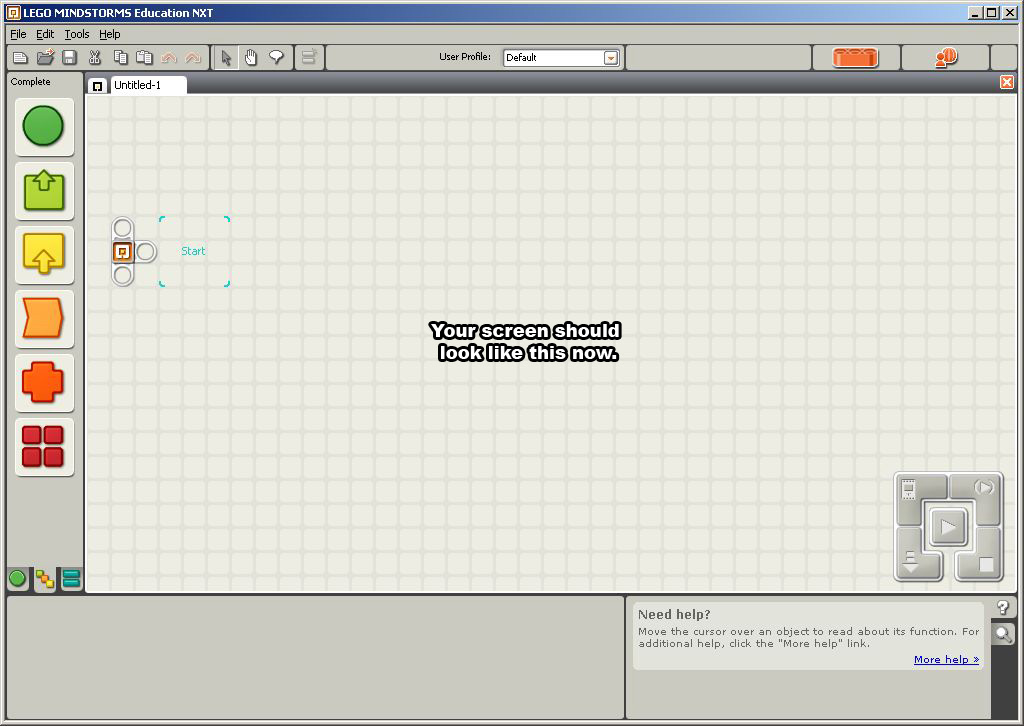7. Click on Actions, then on the Motor icon to select it.
8.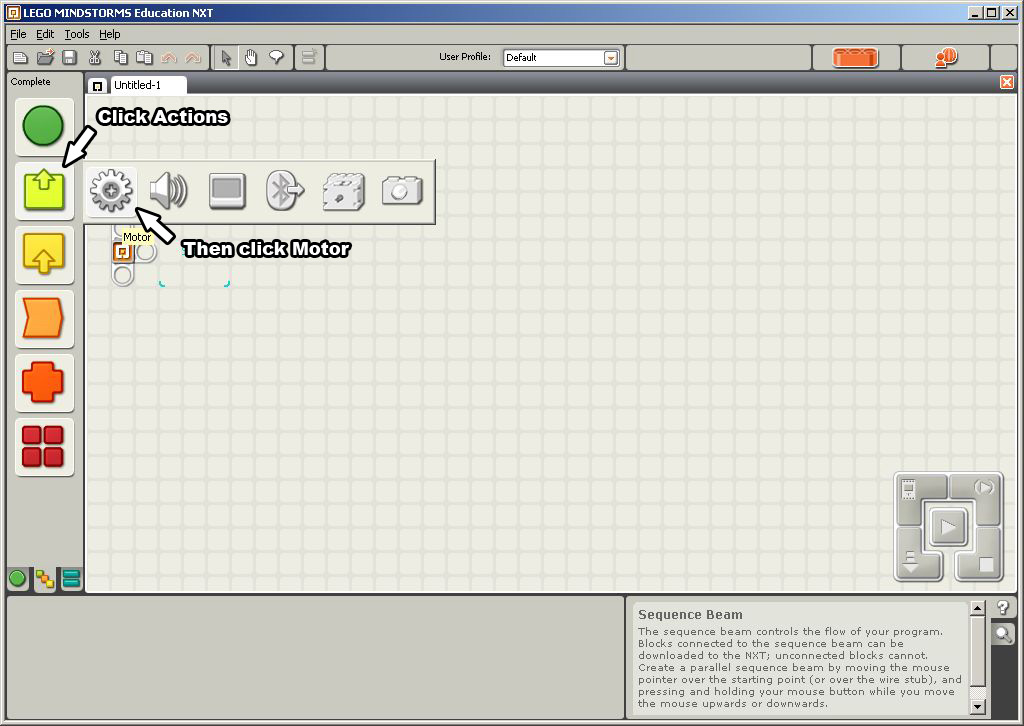9. Place the motor icon on to the "start" square.
10.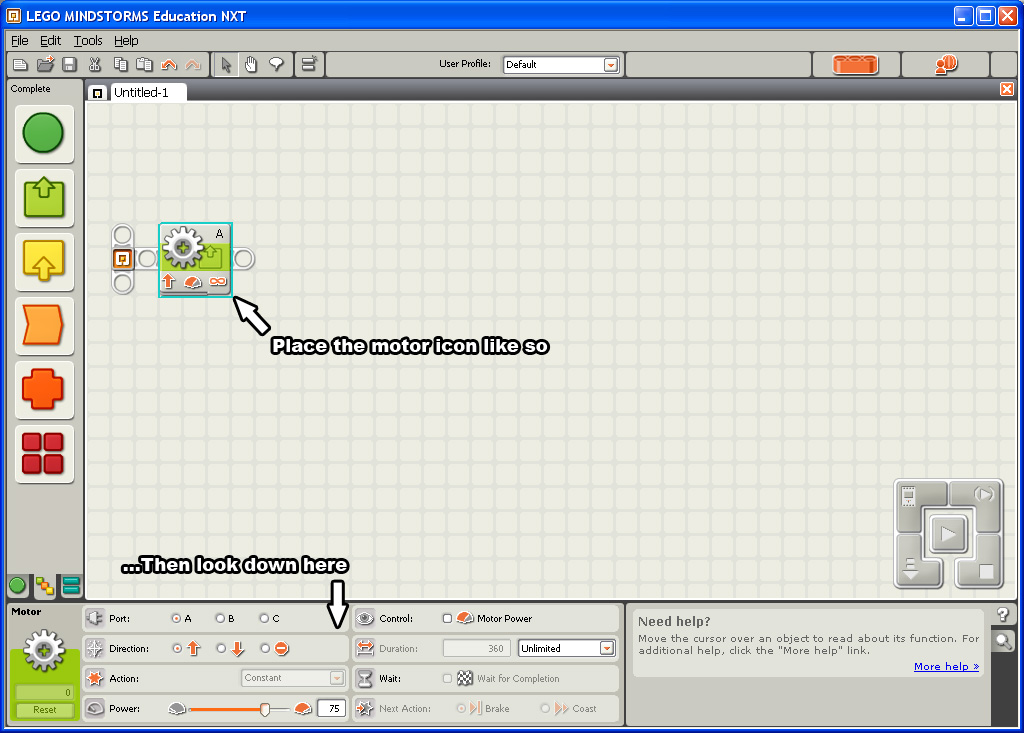11. Click the motor icon to see its properties. Here you can define exactly how you want the motor to spin and when to stop.
12.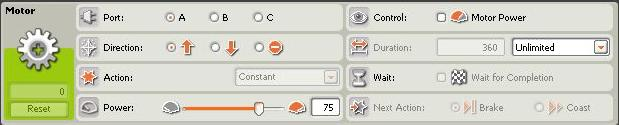13. Continue writing your program by placing icons after each other. The execution of your program will run from left to right.
In the sample program below, motor A will spin forward for 5 seconds, Stop, Wait 5 seconds, and then spin backwards for another 5 seconds.
14.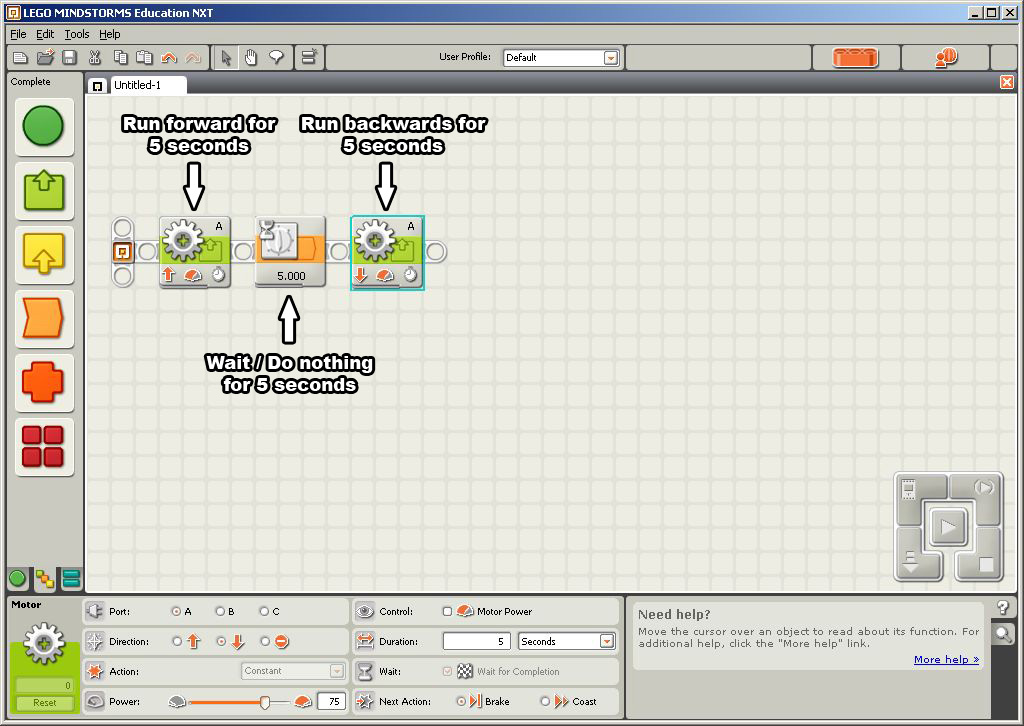15. For this lab you can use the Move icon to easily instruct your robot to move.
16.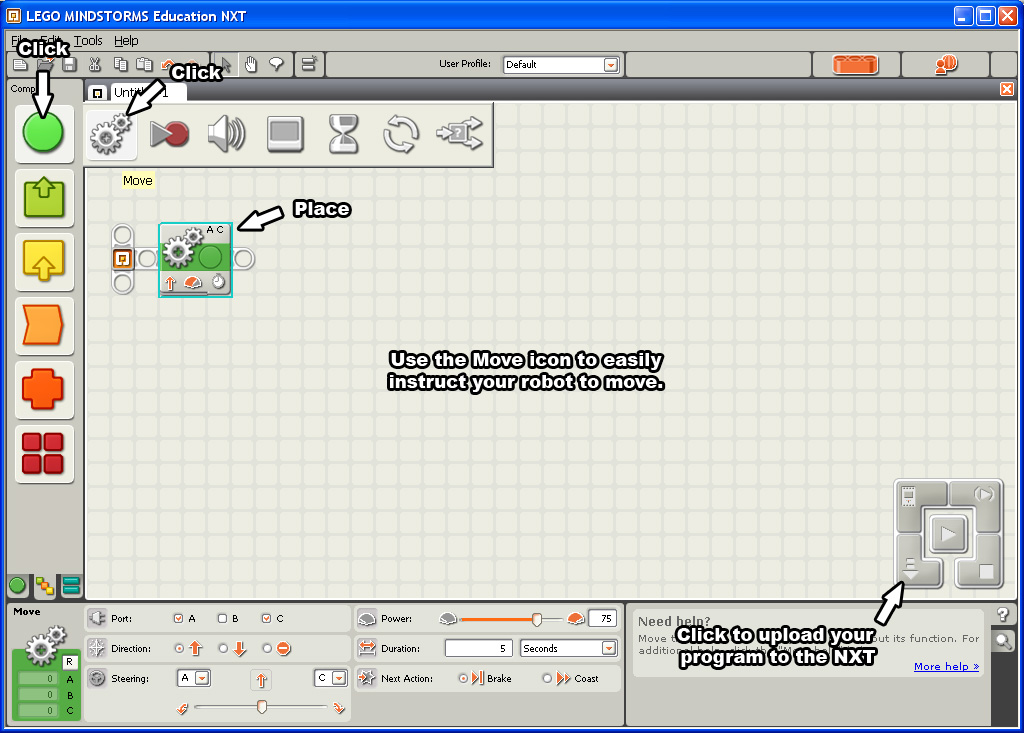17. Whenever you need to, you can use Robot Educator to quickly learn how to program in Lego Mindstorms.
18.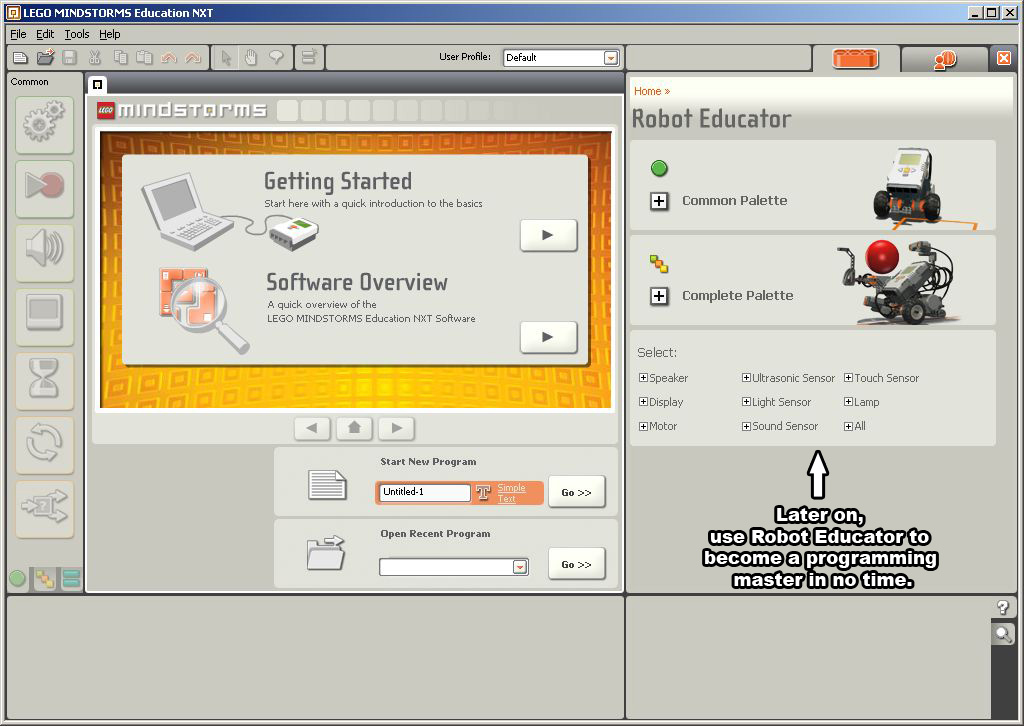Note: Program and test your robot for each test specified. To do this, make a list of the tests in your lab notebook. Brainstorm what you want the robot to do in each test. Then, use your notes to write your program. Record the standard, average, accuracy, and precision. If your robot does not operate correctly, troubleshoot the problem. Your TA must initial your original data.

### Distance Test

Note: Use the Metric system for this test

In this test, the robot will go forward a set distance in a specified time. You are to test the robot's accuracy and precision for this distance. Before testing begins the standard must be found. To find the standard, program the robot to go forward for 5 seconds and measure the distance it travels. Divide your result by 5, and then multiply by 4. This number is your standard. Once the standard has been obtained, the testing can begin.

Warning: Read the section on the Angle of Deviation Test before performing this test.

1. Upload a program that will set the robot to travel for 4 seconds
2. Measure the distance traveled.
3. Repeat this test 10 times.

### Angle of Deviation Test

Note: This test can be performed at the same time as the Distance Test. Since the robot is expected to travel in a straight line, the standard is 360°.

In this test, the robot will move forward for 4 seconds and the angle of deviation will be recorded. The angle of deviation is the angle between a straight line and a line drawn from the starting point to where the robot finishes.

1. Use a protractor to draw a 0° line from the start point.
2. Mark the start point and place your robot there. Identify a reference point on the robot.
3. Run the Distance Test.
4. Make a mark at the point where the robot finished, and draw a line from the start point to this mark.
5. Measure the positive angle of deviation.
6. Repeat this procedure 10 times.

### Rotation Test

In this test, the robot will rotate around an axis. Again, the standard must be obtained. By running the rotation test for 5 seconds and dividing the angle obtained by 5, you will determine how many degrees your robot rotates per second. Finally, you will need to project how many degrees your robot will rotate in 4 seconds. This is the standard.

1. Write the program in Mindstorms to perform this test. Your TA will explain this process.
3. Make a center point on a sheet of paper.
4. Run the program for 5 seconds.
5. Mark the reference point after rotation and measure the rotation angle.
6. Divide this value by 5 to determine the angle of rotation for 1 second.
7. Multiply the angle of rotation per second by 4 to obtain the standard.
8. Go back to the Mindstorms program and change the time from 5 seconds to 4 seconds.
9. Upload the new program to the NXT.
10. Run the program 10 times. Make sure to measure the angle the robot rotated by each time.

You now have measurements for each test you have performed. You must analyze this data. Calculate the average, accuracy, and precision for each test. Compare your results to the standard in order to determine if your robot is accurate and precise. You must also calculate percent accuracy and percent precision. Use this formula to calculate percent accuracy:In this equation, %Acc is the percent accuracy, PS is the standard value, and AP is the value you measured.

### Examples:

1. If PS was determined to be 5cm and AP was found to be 7cm, then the percent accuracy (%Acc) is 60%. This test does not pass the 80% criterion.
2. If PS was determined to be 5 feet and AP was found to be 25 feet, then the result of the equation is -300%. Since a negative percentage has no meaning in engineering, it can be said that the product has a percent accuracy of zero. Any percent accuracy less than zero is considered to be equal to zero.
3. If PS was determined to be 10 ft, and AP was found to be 8 ft, then the result of the equation is exactly 80%, which is a passing result.

Use this formula to calculate % precision:

%Prec = 100e-BP

In this equation, %Prec is the percent precision, B is the exponential decay factor, and P precision you measured. Each individual test requires the use of a different exponential decay factor (B):

Distance Test: B = 0.0972

Angle of Deviation Test: B = 0.0323

Rotation Test: B = 0.0093

### Examples:

1. For the Distance Test, if the precision (P) was measured to be 0.5 cm, the percent precision (%Prec) is 95.3%. This robot passes the 80% criterion for this test.
2. For the Angle of Deviation Test, if the precision (P) was measured to be 10.25°, the percent precision (%Prec) is 71.8%. This robot does not pass the 80% criterion.

### Tabulation of Results

To decide if the product is ready to manufacture, arrange your data in a table designed to calculate its precision and accuracy. Remember it must pass all the tests at a rate of at least 80%.

 Accuracy %Acc Pass/Fail Precision %Prec Pass/Fail Distance Test Angle of Deviation Test Rotational Test

Your lab work is now complete. Please clean up your workstation. Return all unused materials to your TA. Refer to section 3 Your Assignment for the instructions you need to prepare your lab report.

## Footnotes

1 Oakes, W.C., L.L. Leone, and C.G. Gunn, Engineering Your Future w:st="on">MI: Great Lakes Press, 2002